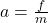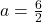## Alex pushes on a 2.0 kg book, resulting in a net force of 6.0 N on the book. What is the acceleration of the book? A. 12 m/s2

Question

Alex pushes on a 2.0 kg book, resulting in a net force of 6.0 N on the book.
What is the acceleration of the book?
A. 12 m/s2
B. 1.5 m/s2
C. 3.0 m/s2
D. 24 m/s2
HELPPPP

in progress 0
2 months 2021-07-21T10:47:01+00:00 1 Answers 1 views 0

## 3.0 m/s²

Explanation:

The acceleration of an object given it’s mass and the force acting on it can be found by using the formulaFrom the question we haveWe have the final answer as

### 3.0 m/s²

Hope this helps you